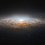# Two Proofs Of The Unique Self-Differentiation Property of $Ce^x$

Ever since I learned elementary calculus, I have been wondering about the proof of the unique self-differentiation of $Ce^x$, $C$ a constant. One of the proofs below I was led to by a calculus textbook; another I figured out by myself.

$1$: Using the Mean Value Theorem

Let $f(x)$ be a function so that $f(x)=f'(x)$. Define a new function

$g(x)=\frac{f(x)}{e^x} (1)$

Now, find $g'(x)$:

$g'(x)=\frac{e^xf'(x)-e^xf(x)}{e^{2x}}$

$g'(x)=\frac{e^x(f'(x)-f(x))}{e^{2x}}$

But our base assumption is that $f(x)=f'(x)$, or that $f'(x)-f(x)=0$. In other words,

$g'(x)=0$

Now, the Mean Value Theorem implies that only constant functions have zero derivative. This means that $g(x)=C$ for some constant $C$. Plugging this into $(1)$ yields:

$C=\frac{f(x)}{e^x}$

$f(x)=Ce^x$

$2$: Using the Taylor Expansion of $e^x$

Note: I would like to thank Alexander Gibson for pointing out that this proof only applies to the set of analytic functions.

Once again, let $f(x)$ be a function so that $f(x)=f'(x)$. Notice that this implies the following:

$f(x)=f'(x)=f''(x)=...=f^{(n)}(x)$

because

$f(x)=f'(x)\implies\lim_{h\to 0} \frac{f(x+h)-f(x)}{h}=\lim_{h\to 0} \frac{f'(x+h)-f'(x)}{h}\implies f'(x)=f''(x)$

and so forth. We can also say that

$f(a)=f'(a)=f''(a)=...=f^{(n)}(a)=C$

for some constant $C$. Use the theory of Taylor series to expand $f(x)$:

$f(x)=f(a)+f'(a)(x-a)+f''(a)\frac{(x-a)^2}{2!}+...+f^{(n)}(a)\frac{(x-a)^n}{n!}+...$

for $f(x)$ around any point $x=a$. Inputting our initial assumption into the Taylor expansion of $f(x)$ yields:

$f(x)=C+C(x)+C\frac{(x-a)^2}{2!}+...+C\frac{(x-a)^n}{n!}+...$

Divide both sides by $C$:

$\frac{f(x)}{C}=1+(x-a)+\frac{(x-a)^2}{2!}+...+\frac{(x-a)^n}{n!}+...$

Notice that the right hand side is the Taylor expansion of $e^x$ about a point $x=a$. We can conclude that

$\frac{f(x)}{C}=e^x$

$f(x)=Ce^x$

Note: It was okay to assume that the Taylor series converges, because it always does in a small interval about $x-a$.

Whew! That was a lot of theory. If you want to share your own proof of the unique self-differentiation property of $Ce^x$, then share it down below in the comments.Note by Andrei Li
2 years, 11 months ago

This discussion board is a place to discuss our Daily Challenges and the math and science related to those challenges. Explanations are more than just a solution — they should explain the steps and thinking strategies that you used to obtain the solution. Comments should further the discussion of math and science.

When posting on Brilliant:

• Use the emojis to react to an explanation, whether you're congratulating a job well done , or just really confused .
• Ask specific questions about the challenge or the steps in somebody's explanation. Well-posed questions can add a lot to the discussion, but posting "I don't understand!" doesn't help anyone.
• Try to contribute something new to the discussion, whether it is an extension, generalization or other idea related to the challenge.

MarkdownAppears as
*italics* or _italics_ italics
**bold** or __bold__ bold
- bulleted- list
• bulleted
• list
1. numbered2. list
1. numbered
2. list
Note: you must add a full line of space before and after lists for them to show up correctly
paragraph 1paragraph 2

paragraph 1

paragraph 2

[example link](https://brilliant.org)example link
> This is a quote
This is a quote
    # I indented these lines
# 4 spaces, and now they show
# up as a code block.

print "hello world"
# I indented these lines
# 4 spaces, and now they show
# up as a code block.

print "hello world"
MathAppears as
Remember to wrap math in $$ ... $$ or $ ... $ to ensure proper formatting.
2 \times 3 $2 \times 3$
2^{34} $2^{34}$
a_{i-1} $a_{i-1}$
\frac{2}{3} $\frac{2}{3}$
\sqrt{2} $\sqrt{2}$
\sum_{i=1}^3 $\sum_{i=1}^3$
\sin \theta $\sin \theta$
\boxed{123} $\boxed{123}$

Sort by:

Here is a derivation for Euler's number, based on the self-differentiation property. This is a less ambitious derivation than the one posted in the note. It doesn't attempt to prove the uniqueness of the self-differentiation property.

Suppose the following is true for some constant $A$ over all $x$.

$\large{\frac{d}{dx} A^x = A^x}$

Evaluate the difference quotient:

$\large{\frac{d}{dx} A^x = \frac{A^{x + dx} - A^x}{dx} = A^x \\ A^{dx} - 1 = dx \\ A = (1 + dx)^{1/dx}}$

The limit of this expression as $dx$ approaches zero is Euler's number.

- 2 years, 11 months ago

It's a clever proof! Not what I was expecting, but it's smart!

- 2 years, 11 months ago

I was attempting something less ambitious (simply a derivation of Euler's number). I have made notes explaining that.

- 2 years, 11 months ago

Okay! No problem. ;)

- 2 years, 11 months ago

$y = \frac{dy}{dx}$

$\frac{1}{y}dy = dx$

$\int \frac{1}{y}dy = \int dx$

$\ln y = x + C_1$

$e^{\ln y} = e^{x + C_1}$

$y = e^{x}e^{C_1}$

$y = Ce^x$

- 2 years, 11 months ago

Toke dey ke? lol.

- 2 years, 11 months ago

The second proof doesn't necessarily follow, you've assumed the taylor series of f(x) converges, or in other words, that f(x) is what's called analytic.Some functions can be infinitely differentiable(called smooth) and still not be analytic, for instance e^(-1/x), which has all its derivatives at 0 equal to 0, and yet it eventually grows to be much bigger than 0.

However, luckily, in complex analysis, this distinction completely dissolves, and it turns out that even if just one derivative exists, the entire function is analytic, which is just one example of why everything is better in the complex case.

- 2 years, 11 months ago

Thank you for your advice! I have generalized my proof for any interval about $a$, where $a$ is any point along the $x$-axis.

- 2 years, 11 months ago

Nope, not even that works, some dastardly functions are not analytic no matter where they are centered around

- 2 years, 11 months ago

(https://en.wikipedia.org/wiki/Non-analyticsmoothfunction)

- 2 years, 11 months ago

Thank you for your advice! I have now included a note that this proof only works for analytic functions.

- 2 years, 11 months ago

Isn't the first proof some sort of a circular argument?

- 2 years, 2 months ago